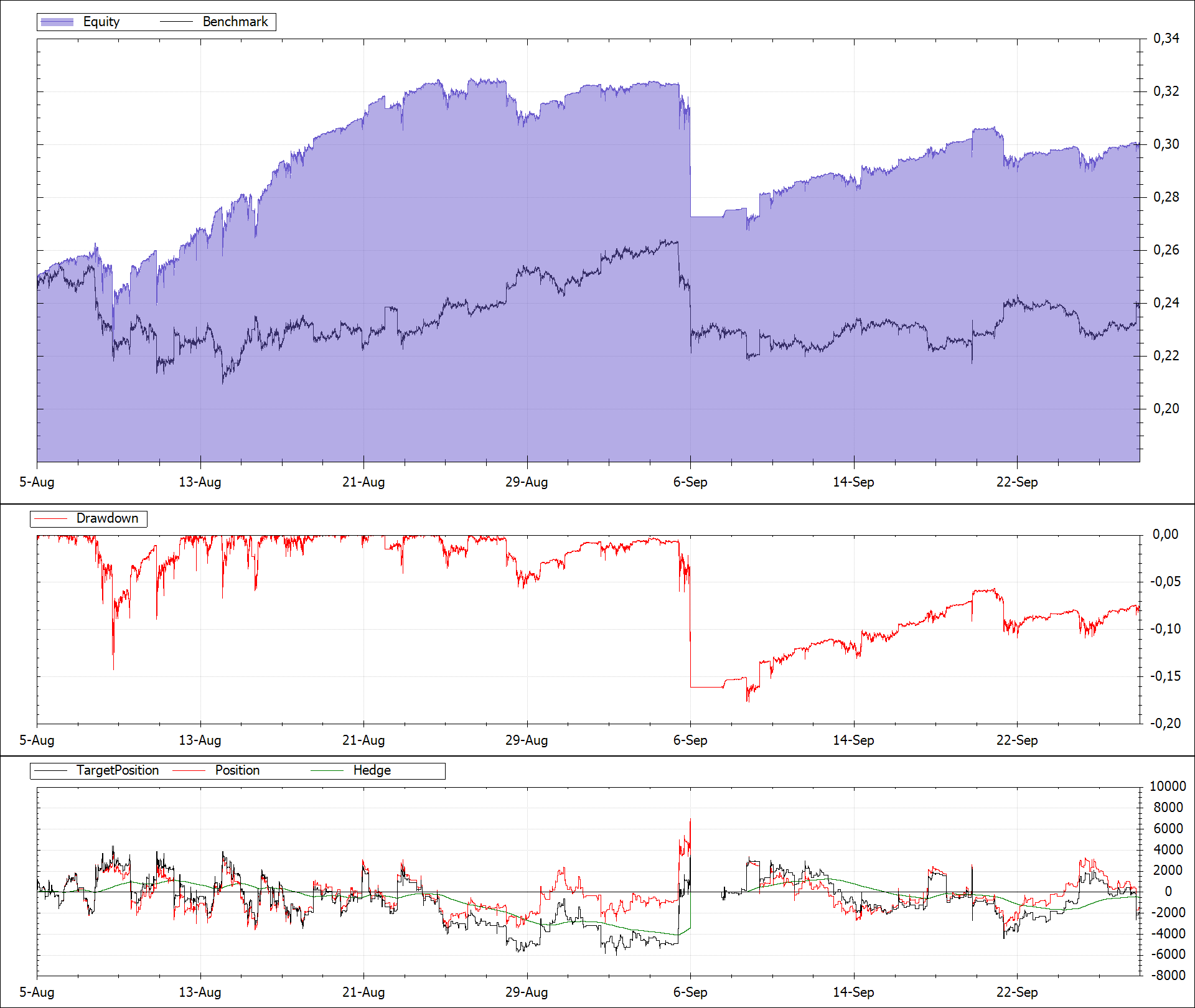I got interested in trading Bitcoins and after reading a paper about market making I implemented a Maket-Making-Bot on the BitMEX exchange.

## Market-Making

A market maker is a liquidity provider who continuously posts both a bid and an ask price using limit orders and waits for them to be filled. When an order gets filled the market makers inventory changes. The market maker profits from the spread between his bid and ask quotes.

There are also risks associated with market making.

There is the inventory risk. When orders get executed the Market-Maker builds an invenroty. If the market makes a strong move in an unfavorable direction while the Market-Maker holds an inventory he will incurr a loss.

There is also the adverse selection risk. This happens when trading against more informed traders. An informed trader only buys from us when he knows the price will rise and he only sells to us when he knows the price will fall. His gain is the Market-Makers loss.

## Research

I read the paper A Learning Market-Maker in the Glosten-Milgrom Model which describes a method to automatically estimate the true value of a traded asset based on the actions that the traders take. It also shows a way to calculate optimal bid and ask prices that also account for the adverse selection risk. The paper has a lot of formulas and initially it seemed a bit complicated but I was able to implement the algorithm using the help of some other online resources.

My C# implementation of the ValueEstimator is here:

``````using MathNet.Numerics.Distributions;
using System.Collections.Generic;

{
public class ValueEstimator
{
private double sigma;
private List<double> values;
private List<double> probabilities;
private List<double> probValue;
private List<double> newProbabilities;
private Normal normal;

private List<double> lookup;
private double lookupStep;
private double lookupShift;

public double TickSize { get; }
public double Alpha { get; set; }

public double Sigma
{
get => sigma;
set
{
sigma = value;
normal = new Normal(0, sigma);
CalculateLookupTable();
}
}

public double Estimate { get; private set; }

public ValueEstimator(double estimate, double tickSize, double alpha, double sigma)
{
TickSize = tickSize;
Alpha = alpha;
Sigma = sigma;

Reset(estimate);
}

public void Update(Side traderSide, double price)
{
for (int i = 0; i < probabilities.Count; i++)
probabilities[i] = newProbabilities[i];
Estimate = DotProduct(values, probabilities);
}

public double CalculateBid()
{
var bid = DotProduct(values, CalculateProbabilities(Side.Sell, Estimate, ref newProbabilities, false));
return bid;
}

{
}

private void Reset(double estimate)
{
values = new List<double>();
probabilities = new List<double>();

for (var v = estimate - 4 * sigma; v <= estimate + 4 * sigma; v += TickSize)
{
probabilities.Add(normal.CumulativeDistribution(v - estimate + TickSize * 0.5) - normal.CumulativeDistribution(v - estimate - TickSize * 0.5));
}

probValue = new List<double>(new double[values.Count]);
newProbabilities = new List<double>(new double[values.Count]);
Estimate = DotProduct(values, probabilities);

CalculateLookupTable();
}

private void CalculateLookupTable()
{
lookupShift = 8 * sigma;
lookupStep = TickSize;
lookup = new List<double>();
for (var v = -8 * sigma; v <= 8 * sigma; v += lookupStep)
}

private List<double> CalculateProbabilities(Side side, double price, ref List<double> newProbabilities, bool allowReset = true)
{
if (allowReset)
{
if (price < values[values.Count / 2] - 3 * Sigma || price > values[values.Count / 2] + 3 * Sigma)
{
//Reset(Estimate);
Reset(price);
}
}

for (int i = 0; i < probValue.Count; i++)
{
// Calculate probabilities for informed traders
var probInf = CumulativeDistribution((int)side * (values[i] - price));

var probUnf = 0.5;

// Joint probability
probValue[i] = (Alpha * probInf) + ((1 - Alpha) * probUnf);
}

// Calculate posterior probability distribution
var prob = DotProduct(probabilities, probValue);
for (int i = 0; i < probabilities.Count; i++)
newProbabilities[i] = probabilities[i] * (probValue[i] / prob);

return newProbabilities;
}

private double DotProduct(List<double> a, List<double> b)
{
var result = 0.0;
for (int i = 0; i < a.Count; i++)
result += a[i] * b[i];
return result;
}

private double CumulativeDistribution(double v)
{
var index = (int)((v + lookupShift) / lookupStep);
if (index < 0 || index >= lookup.Count)
return normal.CumulativeDistribution(v);
return lookup[index];
}
}
}
``````

## Results

I collected quote data from BitMEX and used it for backtesting my Market-Making strategy that uses the above ValueEstimator.

I found out that I could not directly use the bid and ask prices calculated by the algorithm because of the inventory risk. The algorithm would build a large inventory and then the market would move against it resulting in a loss.

I solved the problem by adding a shift value to the calculated prices thus increasing the market makers spread.

I optimized the other parameters like alpha and sigma using my particle swarm optimization framework. Good values for sigma seemed to be around 80 for alpha and 10 for sigma.

The result in the backtest for a period of almost two months looks like this:When a trend develops the market maker stops making profits and potentially also makes losses. When there is a strong move while the market maker holds an inventory there will be large losses. This happend around the 6th September. For this case I have a 15% stoploss built in. When this drawdown limit is reached the bot exits the market for 36 hours.

## Conclusion

Using market making it is possible to make consistent small profits but ocassionally there will be large losses when either a trend develops or the market makes a strong move while holding an inventory.